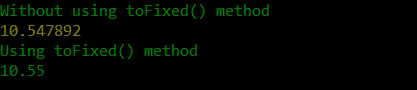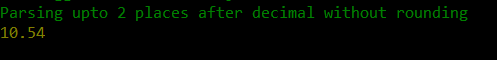# How to parse float with two decimal places in JavaScript ?

• Last Updated : 26 Jul, 2021

JavaScript is high level, dynamically typed, and interpreted client-side scripting language. JavaScript provides an inbuilt parseFloat() method to parse a string and returns a floating-point number.

The parseFloat() method checks if the first character of the string is a number, if it is a number the method parses the string till the end. In case the first character of the string is not a number then parseFloat() returns NaN (Not a Number). The parseFloat() method parses the entire string. If the passed string has 5 digits after the decimal then the output is a floating-point number with 5 digits after the decimal. To limit the number of digits up to 2 places after the decimal, the toFixed() method is used. The toFixed() method rounds up the floating-point number up to 2 places after the decimal.

Syntax:

`parseFloat(string) `

Parameters:

• String: The floating-point number in the string format that is to be parsed.

Return value: It returns the parsed floating-point number if the first character of the string is a number else it returns a NaN.

Syntax:

`toFixed( int )`

Parameter

• int: The number of places after the decimal up to which the string must be parsed.

Return value: It returns the number or string rounded up to the specified places after the decimal. If the specified value is greater than the number of digits after decimal in the actual string then the resulting value is padded with 0 to maintain the number of digits after decimal in the final output.

Example 1:

## Javascript

 `var` `num1 = parseFloat(``"10.547892"``) ` `var` `num2 = parseFloat(``"10.547892"``).toFixed(2) ` `console.log(``"Without using toFixed() method"``); ` `console.log(num1); ` `console.log(``"Using toFixed() method"``); ` `console.log(num2);`

Output:Example 2: The above example rounds up the number up to 2 places after the decimal. But in some cases, the developer may not want to round up the number. This is an alternative method to get the floating-point number up to the specified places without rounding it.

In this example, a function ParseFloat() is defined which takes 2 arguments. The first argument is a string or number to be parsed and the second argument is the number of places after the decimal.

Approach: At first, the first argument is converted to a string, this helps if the passed argument is not already in string format. The string is now sliced from the starting up to the number of places specified after the decimal. Finally, the sliced string is returned after type conversion into a number. The limitation of this method is that unlike parseFloat(), this method cannot detect if the passed string actually contains a floating-point number.

Example:

## Javascript

 `function` `ParseFloat(str,val) { ` `    ``str = str.toString(); ` `    ``str = str.slice(0, (str.indexOf(``"."``)) + val + 1);  ` `    ``return` `Number(str);    ` `} ` `console.log(ParseFloat(``"10.547892"``,2)) `

Output:JavaScript is best known for web page development but it is also used in a variety of non-browser environments. You can learn JavaScript from the ground up by following this JavaScript Tutorial and JavaScript Examples.

My Personal Notes arrow_drop_up
Recommended Articles
Page :